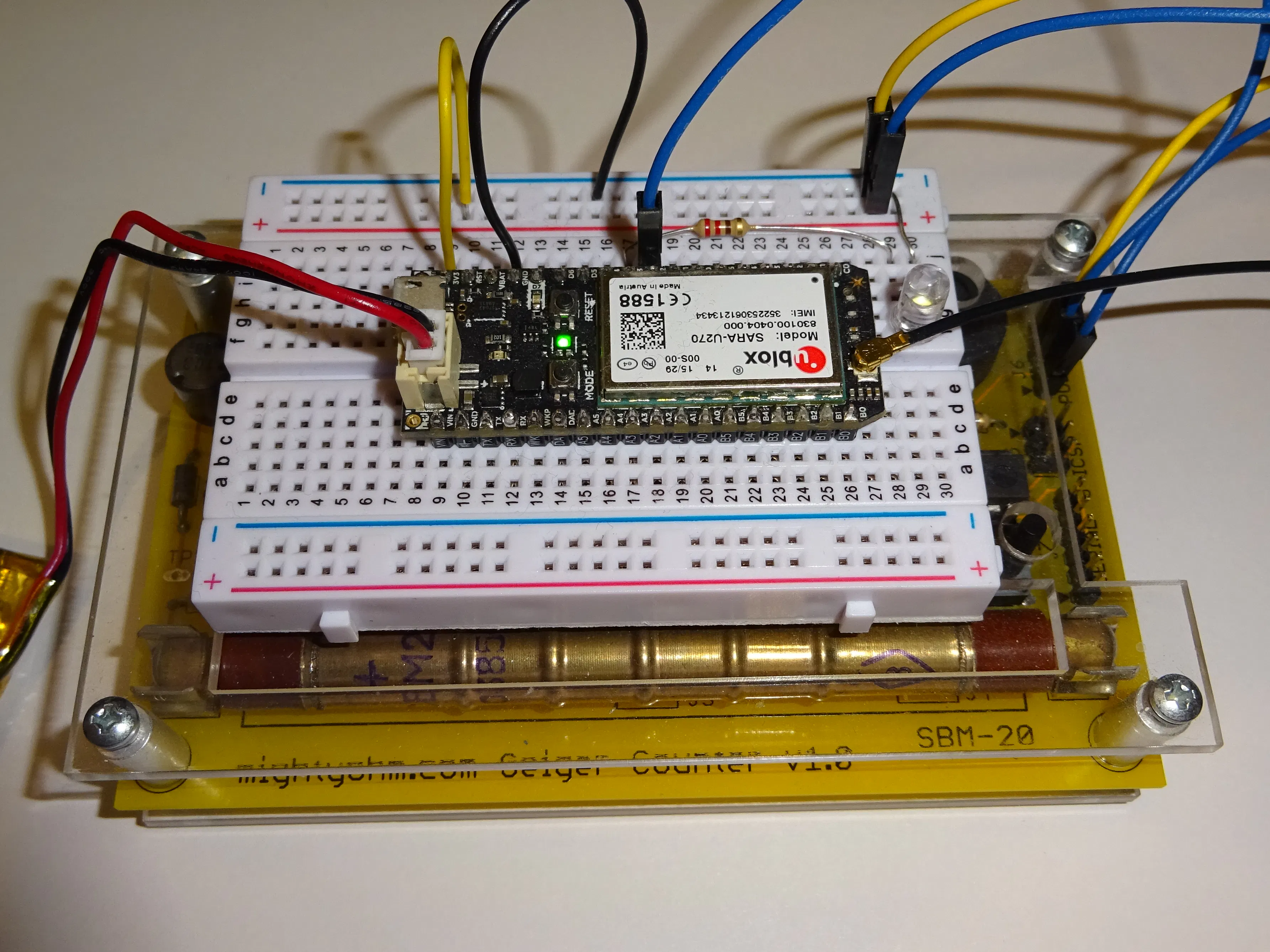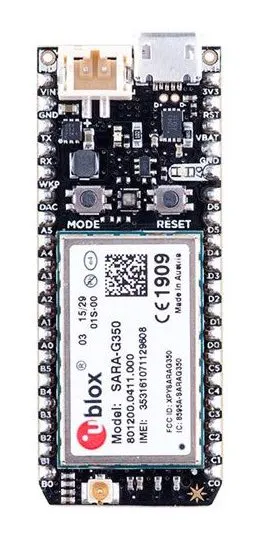Created February 29, 2016 © Apache-2.0

# Internet Geiger Counter

Internet connected Geiger Counter. Either WiFi with a Photon or Cellular with an Electron.

Beginner1 hour234## Things used in this project

### Hardware componentsParticle Photon Either Photon or Electron
×1Particle Electron Either Photon or Electon
×1
 Might Ohm Geiger Counter
×1×1LED (generic)
×1Resistor 1k ohm
×1

### Software apps and online services

 Tinamous

## Code

### Geiger Counter - Electron or Photon.

Arduino
You will need to manually add the PowerShield library in Particles build environment.
```// This #include statement was automatically added by the Particle IDE.
#include "PowerShield/PowerShield.h"

int led = D3;
int pulsePin = D2;
int debugPin = D7;

bool hasBattery = true;

// #if PLATFORM_ID==6      // photon
// Photon specific.
PowerShield batteryMonitor;

// #if PLATFORM_ID==10      // electron
// Electron specific
FuelGauge fuel;

// interrupt/timer driven values.
int volatile countPerInterval = 0;
int volatile ledOn = 0;
bool volatile shuffleAverageCountsPerInterval = false;

int loopDelay = 10;

int lastCountPerInterval = 0;

const int maxPointsPerInterval = 60;
int countsPerInterval[maxPointsPerInterval]; // = {0,0,0,0,0,0};

// Current CPM value readable as a variable
// rather than being published
// so it's available for IFTTT
int cpm = 0;

// 0 = normal
// 1 = raised
// 2 = high

#if PLATFORM_ID==6
// Photon - publish every n seconds
int publishInterval = 10;
#else
// Electorn - publish every n minutes
int publishInterval = 10 * 60;
#endif

// Every second shuffle the buckets and update the average CPM.
Timer updateAveragesTimer(1000, shuffleAverageCountsPerIntervalTick);

// This routine runs only once upon reset
void setup()
{
// Take control of the RGB LED
RGB.control(true);
RGB.brightness(25);
RGB.color(255, 0, 255);

// Initialize pins
pinMode(pulsePin, INPUT_PULLDOWN);
pinMode(led, OUTPUT);
pinMode(debugPin, OUTPUT);
digitalWrite(debugPin, HIGH);

// initialise the averaging array
for (int i  = 0; i<maxPointsPerInterval; i++) {
countsPerInterval[i] =0;
}

// Particle variables to allow reading by IFTTT etc.
Particle.variable("cpm", cpm);

// Setup I2C bus (used for battery monitor)
Wire.begin();

// Start the timers...
updateAveragesTimer.start();

if (hasBattery) {
// Photon.
batteryMonitor.reset();
batteryMonitor.quickStart();
int batteryVersion = batteryMonitor.getVersion();
}

Particle.publish("Status","Geiger setup complete. V1.3.0. Publish Interval: " + String(publishInterval) + "s");

// Once we are ready to start monitoring the radiation, connect up the
// pulse sense pin interrupt.
RGB.color(0, 255, 0);

digitalWrite(debugPin, LOW);
}

// This routine loops forever
void loop()
{
// Switch the radiation LED on if a pulse is detected.
// ledOn value is  decreased until it gets to 0 to allow
// for a longer LED pulse than the loop cycle.
if (ledOn > 0) {
digitalWrite(led, HIGH);
} else {
digitalWrite(led, LOW);
ledOn = 0;
}

// Update the RGB LED for the current radiaiton level

// Ever n seconds shuffle the counter per interval array down one
// to keep a running average and so we can compute the CPM (Counts
// per Minute))
if (shuffleAverageCountsPerInterval) {
doShuffleAverageCountsPerInterval();
shuffleAverageCountsPerInterval = false;
}

// This is set high when the processRadiationTimer timer fires.
}

delay(loopDelay);
ledOn--;
}

// If high level or radiation (well slightly higher than normal)
// make the RGB LED red.
// for intermediate set to yellow,
// Otherwise for low radiation level set the LED to green.

if (cpm > 500) {
Particle.publish("Status", "Warning high radiation level detected!");
}
RGB.color(255, 0, 0);
} else if (cpm > 50) {
}
RGB.color(128, 128, 0);
} else {
}
RGB.color(0, 255, 0);
}
}

// Timer called every 10 seconds

digitalWrite(debugPin, HIGH);

String senml = "{'n':'cpm','v':'" + String(cpm) + "'}";

senml += ",{'n':'cpi','v':'" + String(lastCountPerInterval) + "'}";
senml += ",{'n':'interval','v':'" + String(publishInterval) + "'}";

senml += getPhotonStats();
senml += getElectronStats();

Particle.publish("senml", "{e:[" + senml + "]}");

// Delay to allow the debug pin flash
// to be seen.
delay(250);
digitalWrite(debugPin, LOW);
}

// Get SenML stats about the Electron
// i.e. the battery level, signal stength
String getElectronStats() {

String senml = "";

#if Wiring_Cellular
senml += ",{'n':'qual','v':'" + String(signalStrength.qual) + "'}";
#endif

#if PLATFORM_ID==10
// % of charge (0-100)
float soc = fuel.getSoC();
float vcell = fuel.getVCell();
senml += ",{'n':'vcell','v':'" + String(vcell) + "'}";
senml += ",{'n':'soc','v':'" + String(soc) + "'}";
#endif

return senml;
}

// Get SenML stats about the photon
// i.e. the battery level.
String getPhotonStats() {

String senml = "";

// If photon, use the power shield to get the battery state.
// it might not be repsent.
#if PLATFORM_ID==6

float cellVoltage = batteryMonitor.getVCell();
float stateOfCharge = batteryMonitor.getSoC();

senml += ",{'n':'vcell','v':'" + String(cellVoltage) + "'}";
senml += ",{'n':'soc','v':'" + String(stateOfCharge) + "'}";

#endif

// Devices other than photon use WiFi so
// in case we are one one of them (e.g. P1)
#if Wiring_WiFi
// WiFi signal strength
#endif

return senml;
}

// Once per second shuffle the counts per interval down one
// to give us a 1 minute 60 point average of all the counts.
void doShuffleAverageCountsPerInterval() {

// Compute the current cpm value whilst we are at it.)
// NB: this works because the counterPerInterval array
// is 60 1 second intervals long.
int currentCpm = 0;

// Shuffle average array down to make way for the new point
for (int i=0; i<maxPointsPerInterval-1; i++) {
countsPerInterval[i] = countsPerInterval[i+1];
currentCpm+=countsPerInterval[i];
}

// Store the latest reading in the last array position.
// and reset the value for a new count per interval.
countsPerInterval[maxPointsPerInterval-1] = countPerInterval;
lastCountPerInterval = countPerInterval;
currentCpm+=countPerInterval;
countPerInterval = 0;

// store the current cpm value
// in a variable that
cpm = currentCpm;
}

// Compute the total (sum) of all the counts per second.
// NB: for the first minute the cpm total will be low
// due to 0 average points.
int computeTotalCounts() {
int totalCounts = 0;
for (int i=0; i < maxPointsPerInterval; i++) {
totalCounts+=countsPerInterval[i];
}
}

// ISR.
countPerInterval++;
// How many loops the LED should be on for.
ledOn = 2;
}

}

void shuffleAverageCountsPerIntervalTick() {
shuffleAverageCountsPerInterval = true;
}
```

## Credits

### Stephen Harrison

10 projects • 50 followers
Founder of Tinamous.com, software developer, hardware tinkerer, dyslexic. @TinamousSteve
Thanks to MightOhm.